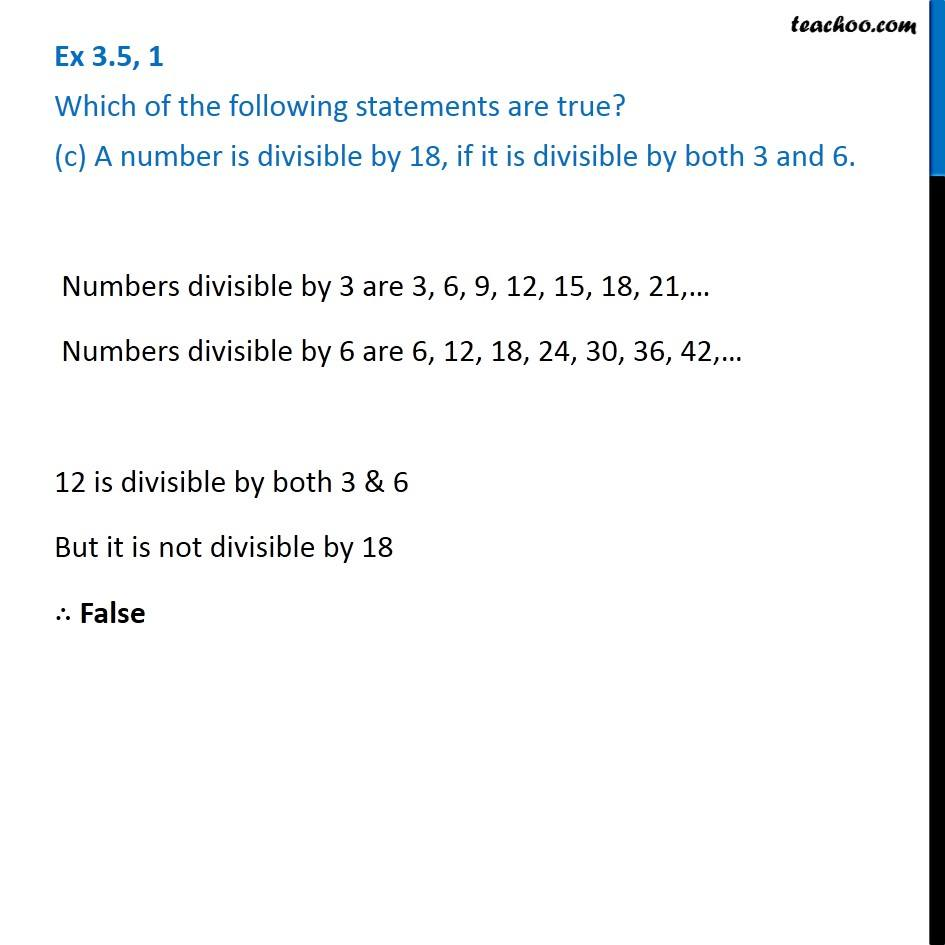Ex 3.5

Chapter 3 Class 6 Playing with Numbers
Serial order wiseGet live Maths 1-on-1 Classs - Class 6 to 12

### Transcript

Ex 3.5, 1 Which of the following statements are true? (c) A number is divisible by 18, if it is divisible by both 3 and 6. We know that If a number is divisible by another number, It is divisible by its factors If a number is divisible by 18, it will be divisible by factors of 18 Factors of 18 are 1, 2, 3, 6, 9, 18 So, number will be divisible by 3 & 6 ∴ True